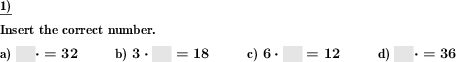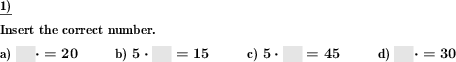Custom math worksheets at your fingertips# Details for problem "Times table fill in blanks"

Quickname: 2776

Elementary School, Primary School, Middle School.

## Summary

Simple times table problems where the product and a factor are given.

## Examples## Description

This problem will develop two main skills, build fact fluency and automaticity in using times table and relationship of multiplication and division. This exercise supports the memorization of multiplication tables. Here the task is not to find the product of multiplication problems from the multiplication tables, but to add the second factor to a known product and a given factor. Thus a division task is to be solved.

An excellent resource to be used as small group work, remediation, quiz, test, drills, unit assessment tool helps to achieve mastery in learning times table. Homeschoolers/ distant learners from grades 3 to 5 will also achieve the following benefits by practicing this worksheet equally.

1. Revise all times table learned.
2. Develop strong concepts and fluency of the times table.
3. Build up the important concepts behind division for future mathematical studies.
4. Calculate mathematical statements for multiplication within the multiplication tables and write them using the multiplication (×), and equals (=) signs.
5. Build real-life skills based on multiplication and division.

Personalized these worksheets to achieve a differentiated level of learners. It can be set whether a certain number series from one to ten is asked or all number series are covered randomly. The factor from the selected number series always comes first. The number of problems can also be set.

The answer key will display all the filled blanks.

Download free printable worksheets for this math problem here. The worksheet contains the problems only, the solution sheet includes the answers. Just click on the respective link.

•Worksheet 1Solution sheet with answers
•Worksheet 2Solution sheet with answers
•Worksheet 3Solution sheet with answers

If you can not see the solution sheets for download, they may be filtered out by an ad blocker that you may have installed. If this is the case, please allow ads for this page and reload the page. The solution sheets will then reappear.

• Do these sample worksheets do not really fit?
• Do you need more math worksheets, with a different level of difficulty?
• Would you like to combine different problems on a worksheet and adjust them to your needs?
• As a teacher, you can put together your own worksheets using the automatically generated math problems provided.
With a free initial credit, you can start creating your own math worksheets in a few minutes.

You can try it for free! Register here, to create custom worksheets now!

## Customization options for this problem

Parameter
Possible values
Number of problems
1, 2, 3, 4, 5, 6, 7, 8, 9, 10
Number to practise
all, 1, 2, 3, 4, 5, 6, 7, 8, 9, 10

## Similar problems

Remark
Description
Also available as a proper division problem
Division problems from the multiplication tables